## 5.7Iterative solution

Imagine a problem with steady ﬂow in which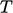is changing at a boundary, e.g. at an inlet. The system could be simulated by solving Eq. (5.12 ) with a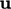ﬁeld which is constant in time.

The simulation would evolve by an iterative sequence which increases timeby an increment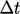, then solves the equation, as shown below.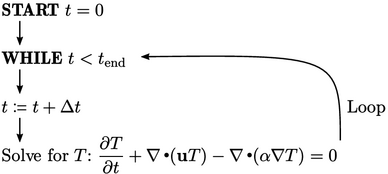### Setting tolerances

Theequation is solved using an iterative method such as Gauss-Seidel. Tolerance parameters determine when the method stops iterating within the current solution step.

To capture the transient solution foraccurately, the equation would need to converge to a suitable absolute tolerance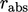at every time step. The question is: what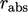is suitable to give an accurate solution? — without setting it to a very small value, e.g.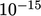, which would would be highly ineﬃcient, due to an excessive number of solver iterations.

Solution accuracy should be determined by some measure, or metric, e.g. temperature rise, pressure drop, drag coeﬃcient, etc., relevant to the objective of the simulation.

The metric should be initially calculated using a value of, e.g.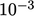. It can then be calculated again at a lower, typically decreasing by one order of magnitude, i.e.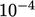.

The metrics are then compared. If the diﬀerence is within the required level of accuracy, thenis optimal. Otherwise,would need to be decreased further and the process repeated.

### Residual calculation at intervals

The residual calculation of Eq. (5.11 ) incurs a signiﬁcant computational cost since it involves multiplications of matrices. That cost can be reduced by setting an interval on the number of sweeps between residual calculations.

The downside is that convergence checks can only happen when the residual is calculated. If the residual is calculated at intervals of every second sweep, a solution that would otherwise converge at an odd number of sweeps is prolonged by a extra sweep.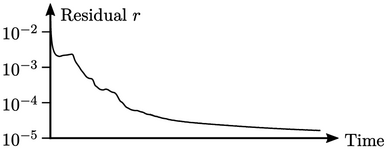Residuals are high when a simulation starts so a relatively high number of sweeps, e.g. 10, may be needed for convergence. The saving of calculating residuals at an interval may then oﬀset the additional cost of unnecessary additional sweeps, giving a lower net cost per time step. However, as the residuals decrease and fewer sweeps are needed, attempts to reduce cost by intermittent residual calculation can be ineﬀective or counter-productive.

Notes on CFD: General Principles - 5.7 Iterative solution